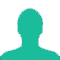# Ajit has a certain average for 9 innings. In the tenth innings, he scores 100 runs thereby increasing his average by 8 runs. His new average is:

A. 20

B. 21

C. 28

D. 32

### Solution(By Examveda Team)

Let Ajit's average be x for 9 innings. So, Ajit scored 9x run in 9 innings.
In the 10th inning, he scored 100 runs then average became (x+8). And he scored (x + 8) × 10 runs in 10 innings.
Now,
\eqalign{ & \Rightarrow 9x + 100 = 10 \times \left( {x + 8} \right) \cr & {\text{or}},\,9x + 100 = 10x + 80 \cr & {\text{or}},\,x = 100 - 80 \cr & {\text{or}},\,x = 20 \cr & {\text{New}}\,{\text{average}} = \left( {x + 8} \right) \cr & \,\,\,\,\,\,\,\,\,\,\,\,\,\,\,\,\,\,\,\,\,\,\,\,\,\,\,\,\,\,\,\,\,\, = 28\,{\text{runs}} \cr}

1.9x8 =72
100-72 = 28 ans new avg

2.Alright

3.Alright

4.Thanks

5.Nice explaination

6.Right

7.Is it right

8.How it becomes (x+8)

9.10.Average= Total or Sum / Number (Total divided by Number)

For 9th inning
x = T/9
9x=T

For 10th inning
x+8=T/10
10(x+8)=T

9x+100=10(x+8)
9x+100=10x+80
x=20

New Average:x+8=28

_____________________________________

Shortcut:

9×8=72
Then, 100-72=28
New Average:28

11.10x8 = 80
100-80 =20
New increment = 20+8

12.Let's do it in shortcut
Assume Till 9th inng his avg will be x
In 10th inng his score was 100 and his total avg increase by x+8
I.e for previous 9 innengs 8 will be given to each inngs
So 9*8 = 72 and for 10th inng it's already 100 so
100-72=28

13.Nice

14.Thanks

15.Awesome app mainly board writing idea 🙂👍

16.Nice sir

17.(9*8)= 72
100-72=28

18.I feel very good. Visit on this app.

19.Salimreza Jim :
100-(8*9)=28
Nice Sir

20.how can this possible?

21.Well awsm app for good method has been

22.Ok, Good.., But when student doing competitive exams we they short cut method, plz explain in short cut methods

23.It's the so best learning website to improve mathematical calculation

24.100-(8*9)=28

25.Good

26.Let, initial average = x
Acq,
10(x+8)-9x = 100
x = 100-80 = 20
New average = 20+8 = 28

27.thanks

28.thanks

29.Good and clear explanation

30.Good Solutions

31.Didn't get it.

32.Let Ajit's average be x for 9 innings. So, Ajit scored 9x run in 9 innings.
In the 10th inning, he scored 100 runs then average became (x+8). And he scored (x+8)*10 runs in 10 innings.
(9x+100)/10 = x+8 (since in 10th inning, 100 more then 9th inning and average gets increses by 8)
therefore on solving this equation we get ,
x=20
But the new average is increased by 8 runs
therefore x+8 i.e 20+8 = 28..

33.Nice solution

34.Can't understand

35.c

36.28

37.Best questions

38.c

39.Its 28

40.28

Related Questions on Average